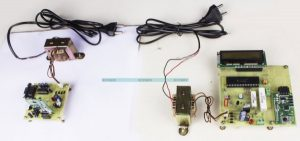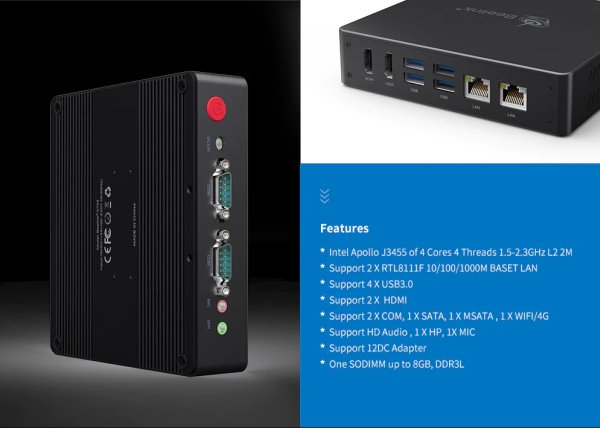# Three phase voltage measurement using pic microcontroller

In our day-to-day life, we consume electrical energy for various purposes such as for powering electrical appliances, gadgets, devices, machines, and so on. So, it is essential to measure the amount of power consumed to generate electricity bill which is usually done by energy meters. In general, AC power is measured using various techniques, here in this article let us discuss about AC power measurement meter using PIC microcontroller.## Power Measurement

The electrical power can be AC power or DC power, energy meter is used for measuring power. There are various types of energy meters, which are classified as digital energy meter, electronic energy meter, watt meter, three phase energy meter, single phase energy meter, AC power measurement meter, and so on.

The AC power is given by the product of RMS voltage value across the load, RMS current across the load, and power factor of the load. This can be represented as shown in the equation below.

Now, AC power measurement can be defined as the measurement of voltage, measurement of current, and measurement of power factor. So, to measure power consumption using PIC microcontroller, it is essential to measure voltage using PIC microcontroller, measure current using PIC microcontroller, and measure power factor using PIC microcontroller.

### AC Voltage Measurement using PIC Microcontroller

The microcontrollers generally operated and manufactured to work with voltage ratings less than or equal to 5V. So, it is not possible to directly measure AC voltage greater than 230V by giving high input voltages to microcontrollers which may cause temporary or permanent damage to microcontrollers.

READ  Up-Down counter on 16*2 LCD using 8051 microcontroller

Hence, it is required to step down the high AC voltage around 230V to 5V for measuring voltage using microcontrollers. The AC voltage measurement using PIC microcontroller can be done using a difference amplifier or potential transformer. The difference amplifier or potential transformer is used to step-down the voltage and then by using analog to digital converter or rectifier the voltage reading is displayed on LCD display.

### AC Current Measurement using PIC Microcontroller

The PIC microcontroller can be used for measuring AC current with the help of difference amplifier, shunt resistor, and analog to digital converter. Shunt resistors are used as transducers for converting current into voltage as microcontroller directly can not read current. Thus, the voltage across the shunt resistor can be measured using PIC microcontroller which is again converted into current using Ohm’s law. Thus, the measured AC current is displayed on LCD display.

### Power Factor Measurement using PIC Microcontroller

The inductor & capacitor cause lagging & leading power factor, current lags voltage by some angle & current leads voltage by some angle respectively. Thus, power factor can be defined as the cosine of the angle between current and voltage and is given as

To measure power factor using PIC microcontroller, the time difference between the voltage and current is determined using zero crossing detection with the help of a microcontroller external interrupt pin. The interrupt is generated by whenever voltage waveform’s zero crossing is detected and internal timer of microcontroller is used for measuring time. Similarly, whenever current waveform interrupt is generated, then the timer stops counting and thus time difference is calculated.

This process repeated for a number of times (say 20 to 30) and the average value is taken for better results. Hence, the time difference is used to determine the phase angle difference between voltage and current. Thus, power factor can be calculated using PIC microcontroller.

Now, by substituting the values of voltage, current, power factor in the above power equation we can measure AC power. The meter used for measuring power factor can be called as power factor meter.

#### Solar Energy Measurement System Conveyed over RF using a PIC Microcontroller

The main aim of this project is solar energy measurement using multiple sensor data acquisition. The project utilizes a solar panel that changes its direction according to sunlight. The solar panel parameters such as intensity of light, temperature, voltage, and current are monitored and also sent to PC using RF.

The project block diagram shown in the above figure consists of various blocks, including solar panel, temperature sensor, light sensor, voltage sensor and current sensor interfaced with the PIC microcontroller. The sensors are used for measuring temperature, light, voltage, and current and is sent to the PC using RF, the same data is displayed over the LCD display.

The power supply block, RF transceiver, PC, max232, 555 timer, and buzzer blocks are connected as shown in the above block diagram. The solar energy measurement can be achieved by measuring the factors such as temperature and light intensity that affect energy generation.

There are various types of meters that include power factor meter, digital energy meter, electronic energy meter, three phase power measurement, energy meter reading over internet, prepaid energy meter with GSM interface, programmable energy meter for electrical load survey.

READ  PIC microcontroller power supply circuit by Chayapol

Are you interested in designing electronics projects using the PIC microcontroller? Then, post your queries or ideas in the comments section below for technical help regarding project solutions.

#### Current Project / Post can also be found using:

• microcontroller programmer project
• circuit diagram for three phase selecting system